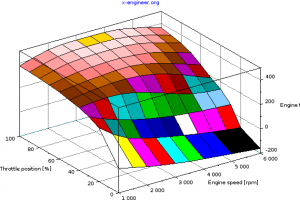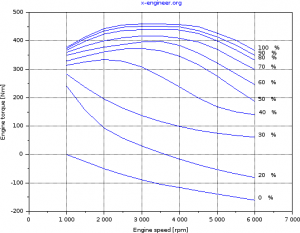# Modeling and simulation of a vehicle with automatic transmission

### 3. Plant model: Engine

The engine used for the simulation example is a Mercedes 5.0 litre V8, with the following performance parameters:

• maximum engine power @ engine speed [kW@rpm]: 225 @ 5600
• maximum engine torque @ engine speed Nm@rpm]: 460 @ 3000-4250

The engine torque is mapped, function of the accelerator (throttle) position and engine speed. Only the full load data points are matched against the real engine torque output. The part load torque values are extrapolated from the full load points.

 Engine torque [Nm] Engine speed [rpm] 1000 1500 2000 2500 3000 3500 4000 4500 5000 5500 6000 Accelerator pedal (throttle) position [%] 0 0 -25 -50 -71 -89 -105 -116 -128 -139 -150 -161 20 241 155 93 57 30 6 -16 -36 -53 -68 -81 30 283 236 195 163 137 116 99 85 75 67 61 40 314 326 334 328 308 275 238 199 168 150 140 50 329 346 361 371 373 365 346 316 276 230 187 60 349 365 378 387 396 398 384 357 322 284 246 70 359 382 400 411 417 417 409 396 370 334 299 80 367 399 424 436 439 440 438 426 400 368 332 90 373 408 435 448 451 451 449 436 414 385 349 100 377 414 443 456 460 460 460 450 427 402 366

In graphical form, the engine torque output at full load and part loads looks like:Image: Engine torque map – 3DImage: Engine torque map – 2D

The engine provides positive torque during acceleration phases and negative (braking) torque in the overrun phases. The engine is modelled as a single inertia lumped parameters and a torque map.

The governing differential equation of the engine model is:

$J_{e} \frac{d \omega_{e} }{dt} = T_{e}-T_{i} \tag{1}$
where:
Te [Nm] – engine torque
Ti [Nm] – impeller torque
Je [kg·m2] – engine and impeller inertia

From equation (1) we can calculate the engine speed as:

$\omega_{e} = \frac{1}{J_{e}} \int (T_{e}-T_{i}) dt \tag{2}$

To convert the engine speed from [rad/s] into [rpm], we use the following expression:

$N_{e} = \frac{30 \cdot \omega_{e}}{\pi} \tag{3}$
where:
Ne [rpm] – engine speed

The engine power can be calculated as:

$P_{e} = \frac{T_{e} \cdot \omega_{e}}{1000} \tag{4}$
where:
Pe [kW] – engine power

Equations (2), (3) and (4) are used to define the Xcos block diagram for engine simulation.

The calculated engine speed is limited to a maximum and a minimum value. The minimum value is considered the idle speed of the engine.

Inputs

 Name Value Description ImplTq_Nm – Impeller torque [Nm] AccrPedlPosn_prc – Accelerator pedal position [%]

Parameters

 Name Value Description EngImplJ_kgm2_C 0.08 Engine and impeller inertia [kg·m2] EngMaxN_rpm_C 7000 Maximum engine speed [rpm] EngMinN_rpm_C 1000 Minimum engine speed [rpm] EngN_rpm_X (table) Engine speed axis [rpm] AccrPedlPosnEng_prc_Y (table) Accelerator pedal position axis [%] EngTq_Nm_Z (table) Engine torque map [Nm]

Outputs

 Name Value Description EngN_rpm – Engine speed [rpm]